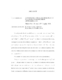## Asymptotic Problems Related to Smoluchowski-Kramers Approximation##### Files
umi-umd-3634.pdf(401.6 KB)
According to the Smoluchowski-Kramers approximation, the solution \qu , also referred to as Physical&quot; Brownian motion, of the Langevin's equation $\mu\ddot{q}_{t}^{\mu,\varepsilon}=-\dot{q}_{t}^{\mu,\varepsilon}+b(q_{t}^{\mu,\varepsilon})+ \sqrt{\varepsilon}\sigma(q_{t}^{\mu,\varepsilon})\dot{W}_{t},\ q_{0}^{\mu,\varepsilon}=q,\ \dot{q}_{0}^{\mu,\varepsilon}=p$, where $\dot{W}_t$\ is Gaussian white noise, converges to solution of the diffusion equation $\dot{q}_{t}^{\varepsilon}=b(\qe)+\sqrt{\varepsilon}\sigma(\qe) \dot{W}_{t},\ q_{0}^{\varepsilon}=q$\ as $\mu\downarrow 0$\ uniformly on any finite time interval for each fixed $\ve&gt;0$. This is the main justification for describing the small particle motion by a diffusion equation. However, this relation is not sufficient for asymptotic problems when some parameter, say $\ve$, approaches 0. We consider two asymptotic problems related to this approximation. First, we study relations between large deviations for these processes \qu\ and \q\ as $\ve\downarrow 0$. In particular, we consider exit problems where relations between asymptotic exit position, asymptotic mean exit time and some other characteristics of the first exit of the trajectories \qu\ and \q\ from a bounded domain are of interest. Under the framework of Freidlin-Wentzell, these asymptotics can be represented by quasi-potential, defined as the infimum of action functional over some set. Action functional and quasi-potentials for \qu\ are calculated in this paper. We establish that the asymptotics of \qu\ and \q\ are close for small particles when $0&lt;\mu\ll 1$. We pay special attention to the case when $b(q)$\ is linear. Then the quasi-potentials can be calculated explicitly and they coincide for \qu\ and \q. Second, we study the wavefront propagation for reaction-diffusion equations with diffusion governed by the infinitesimal generator of process \qu\ and \q\ and reaction term governed by a nonlinear function of KPP-type. In this case, the reaction-diffusion equation related to the process \qu\ is degenerate in terms of variable $(p,q)$. When the diffusion coefficient and nonlinear term are space dependent but only changing slowly in space, we know as $t\rightarrow \infty$, the solution of the reaction-diffusion equation related to the process \q\ behaves like a running wave. Characterization of the position of wavefront for equations related to \q\ is well studied. In this work, we identify two characterizations of the position of the wavefront for the degenerate reaction-diffusion equation related to the process \qu. We analyze two cases, under which we can obtain the convergence of the wavefronts of the degenerate reaction-diffusion equation related to \qu to those of the non-degenerate one related to \q, for small $\mu&gt;0$.